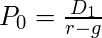## Fun with the Gordon Growth Model

May 182011
##### Thinking about Valuation and Mean-Reversion in the Context of a Simple Discounted Cash Flow Model

The Gordon growth model is a simple discounted cash flow (DCF) model which can be used to value a stock, mutual fund, or even the entire stock market.  The model is named after Myron Gordon who first published the model in 1959.

The Gordon model assumes that a financial security pays a periodic dividend (D) which grows at a constant rate (g). These growing dividend payments are assumed to continue forever. The future dividend payments are discounted at the required rate of return (r) to find the price (P) for the stock or fund.

Under these simple assumptions, the price of the security is given by this equation:In this equation, I’ve used the “0” subscript on the price (P) and the “1” subscript on the dividend (D) to indicate that the price is calculated at time zero and the dividend is the expected dividend at the end of period one. However, the equation is commonly written with these subscripts omitted.

Obviously, the assumptions built into this model are overly simplistic for many real-world valuation problems. Many companies pay no dividends, and, for those that do, we may expect changing payout ratios or growth rates as the business matures.

Despite these limitations, I believe spending some time experimenting with the Gordon model can help develop intuition about the relationship between valuation and return.